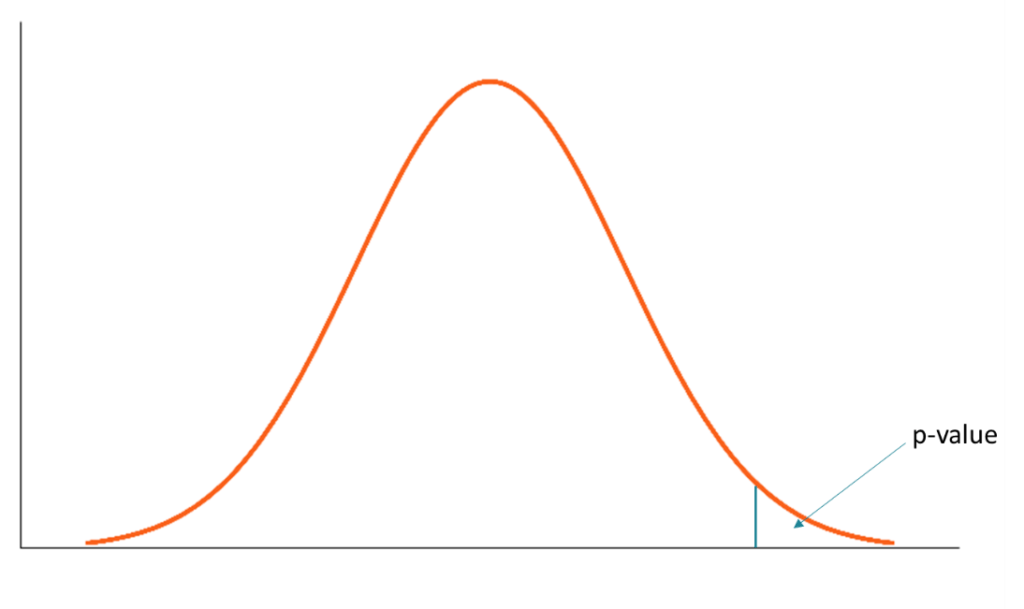# P-value

A probability measure of finding the observed, or more extreme results, when the null hypothesis of a given statistical test is true

## What is the P-value?

In statistical hypothesis testing, the p-value (probability value) is a probability measure of finding the observed, or more extreme results, when the null hypothesis of a given statistical test is true. The p-value is a primary value used to quantify the statistical significance of the results of a hypothesis test.The main interpretation of the p-value is whether there’s enough evidence to reject the null hypothesis. If the p-value is reasonably low (less than the level of significance), we can state that there is enough evidence to reject the null hypothesis. Otherwise, we should not reject the null hypothesis.

The conclusions about the hypothesis test are drawn when the p-value of a test is compared against the level of significance, which plays the role of a benchmark. The most typical levels of significance are 0.10, 0.05, and 0.01, while the level of significance of 0.05 is considered conventional.

### How to Use P-value in Hypothesis Testing?

In order to use the p-value in a hypothesis testing, follow the next steps below:

1. Determine your level of significance (α). The level of significance generally should be chosen during the first steps of the design of a hypothesis test. The most common levels of significance include 0.10, 0.05, and 0.01.
2. Calculate the p-value. There are numerous software applications that offer the calculations. For instance, Microsoft Excel allows the calculation of the p-value using the Data Analysis ToolPak.
3. Compare the obtained p-value with the level of significance (α) and draw the relevant conclusions. The general rule here is if the figure is less than the level of significance, then there is sufficient evidence to reject the null hypothesis of an experiment.

The degree of statistical significance generally varies depending on the level of significance. For example, a p-value that is less than 0.05 is considered statistically significant while a figure that is less than 0.01 is viewed as highly statistically significant.

### Misinterpretations of the P-value

In statistics, the p-value can be truly considered as one of the most commonly misinterpreted concepts. The biggest misconception about the concept is that it is a probability that the null hypothesis is true (or it is a probability that the alternative hypothesis is false).

In reality, the p-value does not determine the probability of the null hypothesis to be true but merely indicates the probability of encountering the results of a study at least as extreme as the actually observed results from if the null hypothesis is true. In other words, it indicates the probability of having enough evidence to reject or not to reject the null hypothesis.